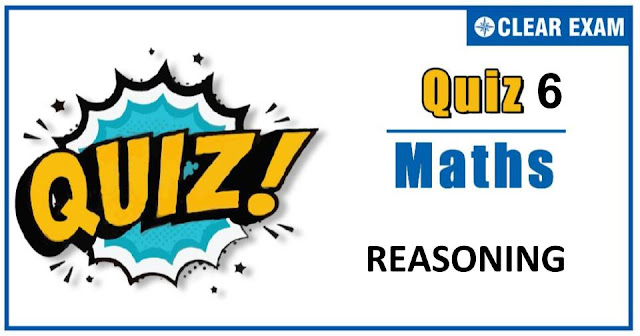## MATHEMATICS REASONING QUIZ-6

As per analysis for previous years, it has been observed that students preparing for JEE MAINS find Mathematics out of all the sections to be complex to handle and the majority of them are not able to comprehend the reason behind it. This problem arises especially because these aspirants appearing for the examination are more inclined to have a keen interest in Mathematics due to their ENGINEERING background.

Furthermore, sections such as Mathematics are dominantly based on theories, laws, numerical in comparison to a section of Engineering which is more of fact-based, Physics, and includes substantial explanations. By using the table given below, you easily and directly access to the topics and respective links of MCQs. Moreover, to make learning smooth and efficient, all the questions come with their supportive solutions to make utilization of time even more productive. Students will be covered for all their studies as the topics are available from basics to even the most advanced.

Q1. ∼(p∨q)∨(∼p∧q) is logically equivalent to
•  ∼p
•  p
•  q
•  ∼q
Solution
∼(p∨q)∨(∼p∧q) ≅(∼p∧∼q)∨(∼p∧q) ≅∼p∧(∼q∨q)≅∼p∨t≅∼p

Q2.If S(p,q,r)=(~P)∨[~(q∧r)] is a compound statement, then S(~p,~q,~r) is :
•  ~S(p,q,r)
•  S(p,q,r)
•  p∨(q∧r)
•  p∨(q∨r)
Solution
S(p,q,r)=(~p)∨[∼(q∧r)] =(∼p)∨[∼q∨∼r] ⇒S (~p,~q,~r)=p∨(q∨r)

Q3.  What are the truth values of (∼p⇒∼q) and ∼(∼p⇒q) respectively, when p and q always speak true in any argument?
•   T,T
•  F,F
•  T,F
•  F,T

Q4. Which of the following propositions is a contradiction?
•  (~p∨∼q)∨(p∼q)
•  (p→q)∨(p∧∼q)
•  (~p∧q)∧(∼q)
•  (∼p∧q)∨(∼q)
Solution
(∼p∧q)∧∼q=∼p∧(q∧∼q)=∼p∧c=c

Q5.Which is a statement?
•  x+1=6
•  5∈N
•  x+y<12
•  None of these

Q6. If p and q are two propositions, then ∼(p↔q) is
•  ∼p∧∼q
•  ∼p∨∼q
•  (p∧∼q)∨(∼p∧q)
•  None of these
Solution
We know that p→q≅∼p∨q and q→p≅∼q∨p ∴p↔q≅(∼p∨q)∧(∼q∨p) ∼(p↔q)≅∼(∼p∨q)∨∼(∼q∨p) ∼(p↔q)≅(p∧∼q)∨(q∧∼p))

Q7.The negation of the proposition q∨∼(p∧r) is
•  ∼q∨(p∧r)
•  ∼q∧(p∧r)
•  ∼p∨∼q∨∼r
•  None of these
Solution
The negation of q∨∼(p∧r) is given by ∼{q∨∼(p∧r)}≅∼q∧(p∧r)

Q8.The statement p⇒p∨q is
•  A tautology
•  Both a tautology and contradiction
•  Neither a tautology nor a contradiction

Q9.Which of the following is not a proposition?
•  3 is prime
•  √2 is irrational
•  Mathematics is interesting
•  5 is an even integer
Solution
Mathematics is interestring is not a proposition.

Q10. Let p and q be two statements, then (p∨q)∨∼p is
•  Tautology
•  Both (a) and (b)
•  None of these
Solution#### Written by: AUTHORNAME

AUTHORDESCRIPTION## Want to know more

Please fill in the details below:

## Latest NEET Articles\$type=three\$c=3\$author=hide\$comment=hide\$rm=hide\$date=hide\$snippet=hide

Name

ltr
item
BEST NEET COACHING CENTER | BEST IIT JEE COACHING INSTITUTE | BEST NEET & IIT JEE COACHING: REASONING-QUIZ-6
REASONING-QUIZ-6
https://1.bp.blogspot.com/-92Dhe0Gh92E/X3k4jzFY1RI/AAAAAAAAAaA/-kWrgqtGbFoqfDsaeHUpNZWm9UVeo98zACLcBGAsYHQ/w640-h336/Quiz%2BImage%2BTemplate%2B%25283%2529.jpg
https://1.bp.blogspot.com/-92Dhe0Gh92E/X3k4jzFY1RI/AAAAAAAAAaA/-kWrgqtGbFoqfDsaeHUpNZWm9UVeo98zACLcBGAsYHQ/s72-w640-c-h336/Quiz%2BImage%2BTemplate%2B%25283%2529.jpg
BEST NEET COACHING CENTER | BEST IIT JEE COACHING INSTITUTE | BEST NEET & IIT JEE COACHING
https://www.cleariitmedical.com/2020/10/REASONING-QUIZ-6.html
https://www.cleariitmedical.com/
https://www.cleariitmedical.com/
https://www.cleariitmedical.com/2020/10/REASONING-QUIZ-6.html
true
7783647550433378923
UTF-8

STAY CONNECTED